PSABPQFJ - Practice---Acc7.3 Lesson 3 Reasoning about Equations with Tape Diagrams (7.EE.B.3)
Part A)

Draw a tape diagram to match the equation.If you do not have the ability to upload an image of your work type "Diagram is on paper."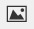Part B)

Draw a tape diagram to match the equation.If you do not have the ability to upload an image of your work type "Diagram is on paper."Select all the equations that match the tape diagram.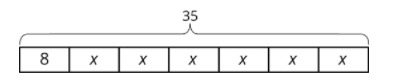Select all that apply:

Point B has coordinates (-2,-5). After a translation 4 units down, a reflection across the y-axis, and a translation 6 units up, what are the coordinates of the image?

Enter your answer as x,y. For example, (3,2) would be entered as 3,2

Part A)

Figure 2 is a scaled copy of Figure 1.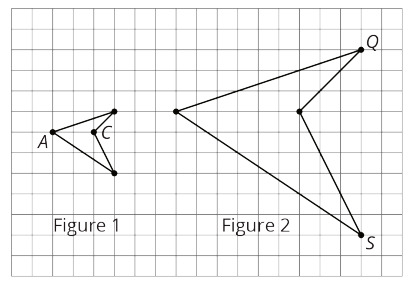Identify the points in Figure 2 that correspond to the points A and C in Figure 1. Label them P and R.

Take a picture of your work and upload using the insert image icon.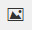If you don't have the ability to insert an image, respond with work is on paper.

Part B)

Figure 2 is a scaled copy of Figure 1.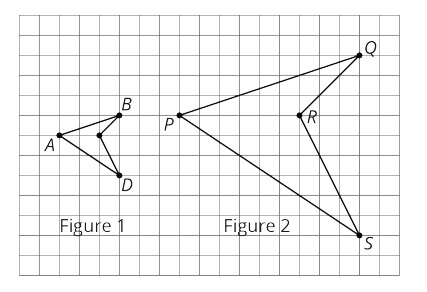What is the distance between P and R?

Type your answer below as a number (example: 5, 3.1, 4 1/2, or 3/2):
Part C)

Figure 2 is a scaled copy of Figure 1.Identify the points in Figure 1 that correspond to the points Q and S in Figure 2. Label them B and D.

Take a picture of your work and upload using the insert image icon.If you don't have the ability to insert an image, respond with work is on paper.

Part D)

Figure 2 is a scaled copy of Figure 1.What is the distance between B and D?

Type your answer below as a number (example: 5, 3.1, 4 1/2, or 3/2):
Part E)

What is the scale factor that takes Figure 1 to Figure 2?Type your answer below as a number (example: 5, 3.1, 4 1/2, or 3/2):
Part F)

G and H are two points on Figure 1, but they are not shown. The distance between G and H is 1. What is the distance between the corresponding points on Figure 2?